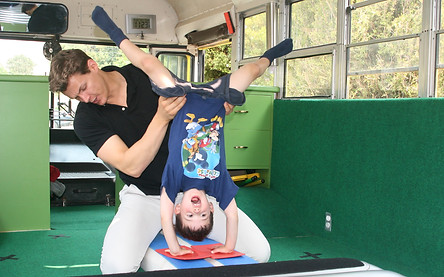Tuition:

Private pod - groups:

###### ​

School groups/pods:

###### The rate for school group/pod lessons is \$74.00 per person per for a 4 week session, with 30-minute lessons. Each group will have a maximum of 8 participants.

***In the event less than 5 students register for class, instead of canceling the program, class time will be as follows:

1 student   = 10 minute  lesson

2 students = 15 minute lesson

3 students = 20 minute lesson

4 students = 25 minute lesson

5 students or more = 30 minute lesson